# Decision Making Decision Making Problem Solving 1 2

• Slides: 14Decision Making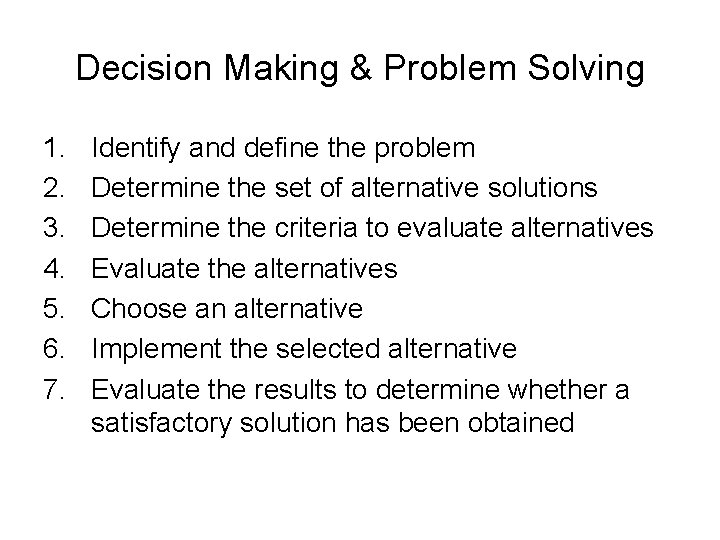Decision Making & Problem Solving 1. 2. 3. 4. 5. 6. 7. Identify and define the problem Determine the set of alternative solutions Determine the criteria to evaluate alternatives Evaluate the alternatives Choose an alternative Implement the selected alternative Evaluate the results to determine whether a satisfactory solution has been obtainedDecision Making The first five steps of Problem Solving constitute the Decision Making process: i. e. : 1. 2. 3. 4. 5. Identify and define the problem Determine the alternatives Determine the criteria Evaluate the alternatives Choose an alternative The choice of an alternative is the Decision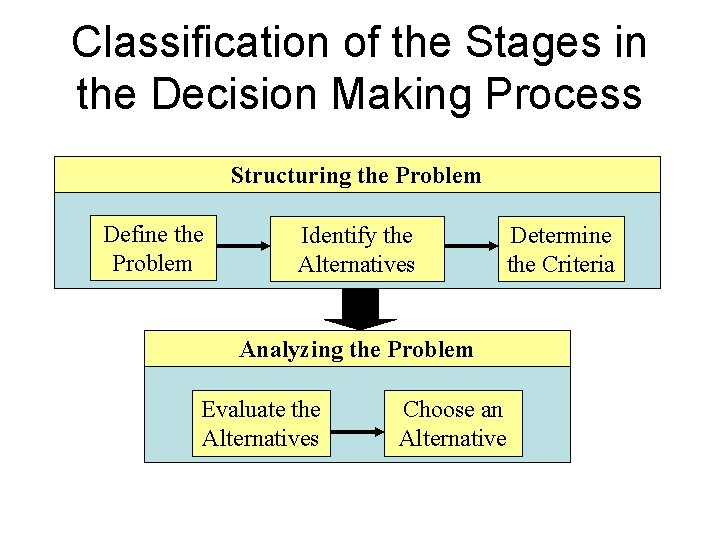Classification of the Stages in the Decision Making Process Structuring the Problem Define the Problem Identify the Alternatives Determine the Criteria Analyzing the Problem Evaluate the Alternatives Choose an Alternative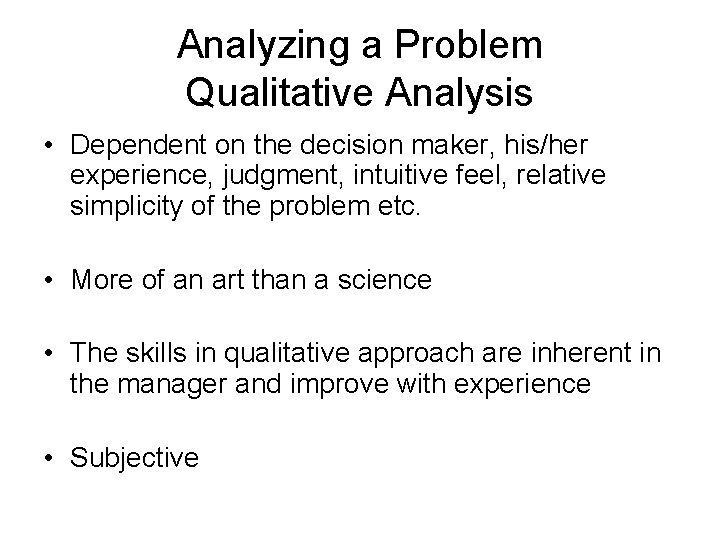Analyzing a Problem Qualitative Analysis • Dependent on the decision maker, his/her experience, judgment, intuitive feel, relative simplicity of the problem etc. • More of an art than a science • The skills in qualitative approach are inherent in the manager and improve with experience • SubjectiveAnalyzing a Problem Quantitative Analysis • Relatively large/complex problem or when the manager lacks previous experience with similar situation or where the nature of the problem demands quantitative treatment • The skills in quantitative approach are learnt only by studying the methods of Management Science • ObjectiveQualitative and Quantitative Analyses have their roles in Decision Making Analyzing the Problem Qualitative Analysis Quantitative Analysis Summary and Evaluation Make the Decision A manager who knows quantitative procedures is in a much better position to compare and evaluate the qualitative and quantitative recommendations and combine them to arrive at the best decision.Summary of the instances requiring Quantitative Analysis • The problem is large or complex; the manager cannot develop a good solution without quantitative analysis • The problem is important; demands a thorough analysis • The problem is new; no previous experience to rely on • The problem is repetitive; quantitative methods save time and effort for the decision maker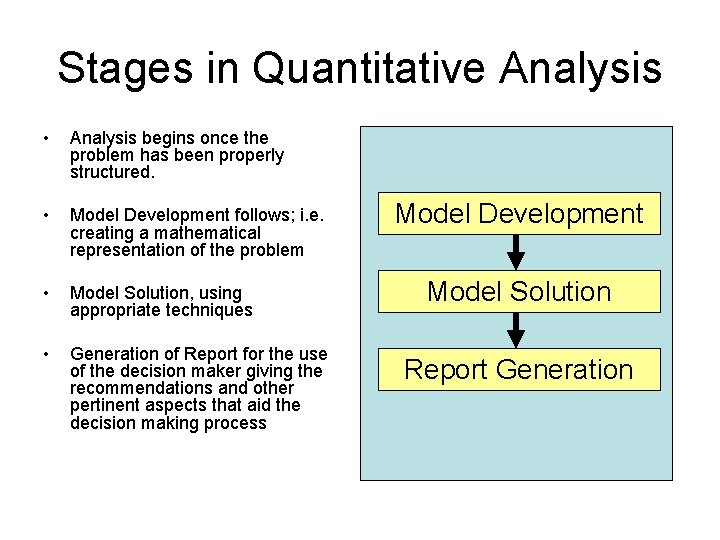Stages in Quantitative Analysis • Analysis begins once the problem has been properly structured. • Model Development follows; i. e. creating a mathematical representation of the problem • Model Solution, using appropriate techniques • Generation of Report for the use of the decision maker giving the recommendations and other pertinent aspects that aid the decision making process Model Development Model Solution Report Generation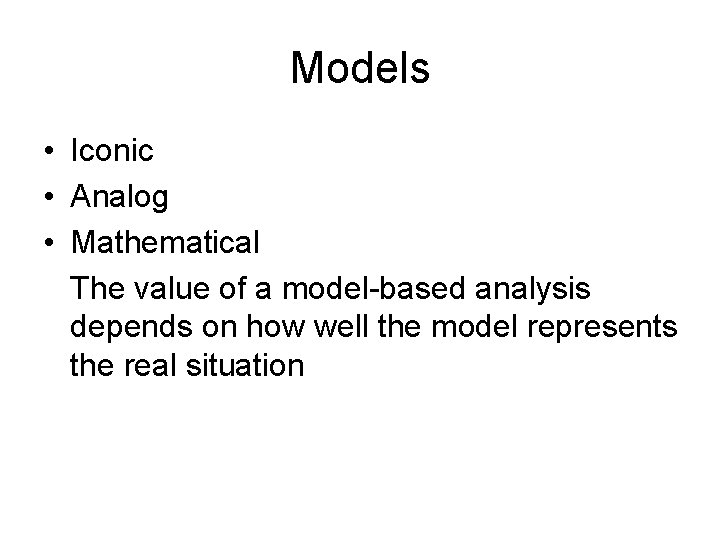Models • Iconic • Analog • Mathematical The value of a model-based analysis depends on how well the model represents the real situationMathematical Models • Objective function: A mathematical function that describes the problem’s objective • Constraint Restrictions that limit the degree to which the objective can be pursued (production capacities, availability of inputs, committed outputs etc. ) • Uncontrollable inputs Environmental factors affecting the objective function and the constraints, but beyond the control of the decision maker • Controllable inputs or decision variable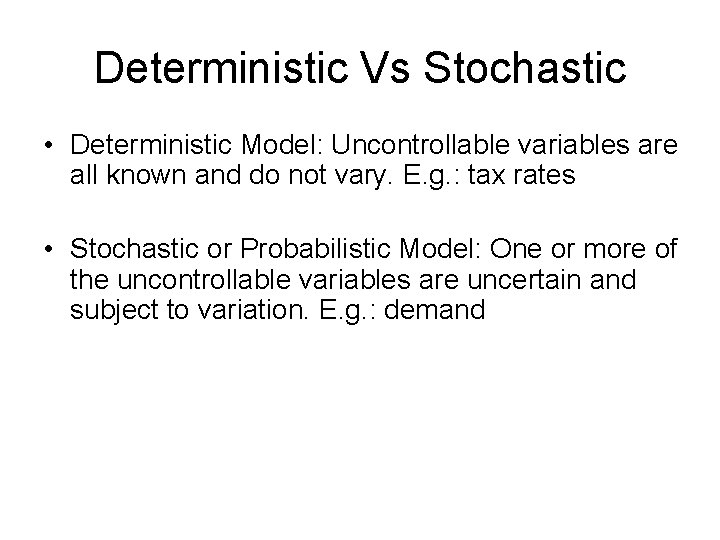Deterministic Vs Stochastic • Deterministic Model: Uncontrollable variables are all known and do not vary. E. g. : tax rates • Stochastic or Probabilistic Model: One or more of the uncontrollable variables are uncertain and subject to variation. E. g. : demand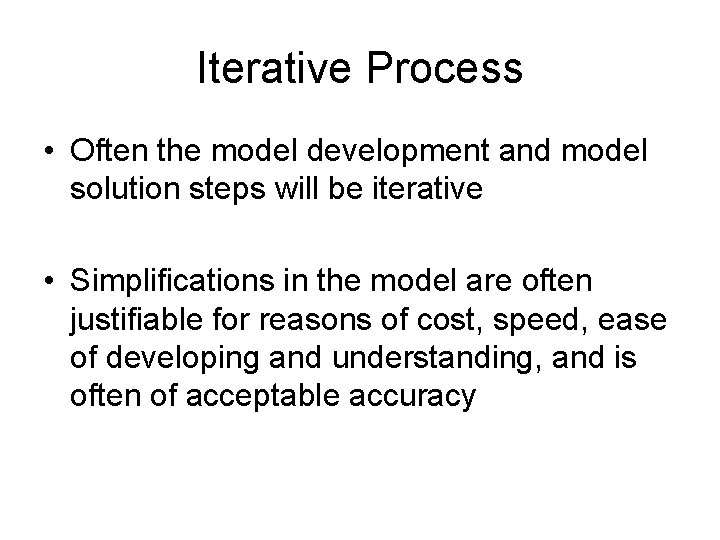Iterative Process • Often the model development and model solution steps will be iterative • Simplifications in the model are often justifiable for reasons of cost, speed, ease of developing and understanding, and is often of acceptable accuracy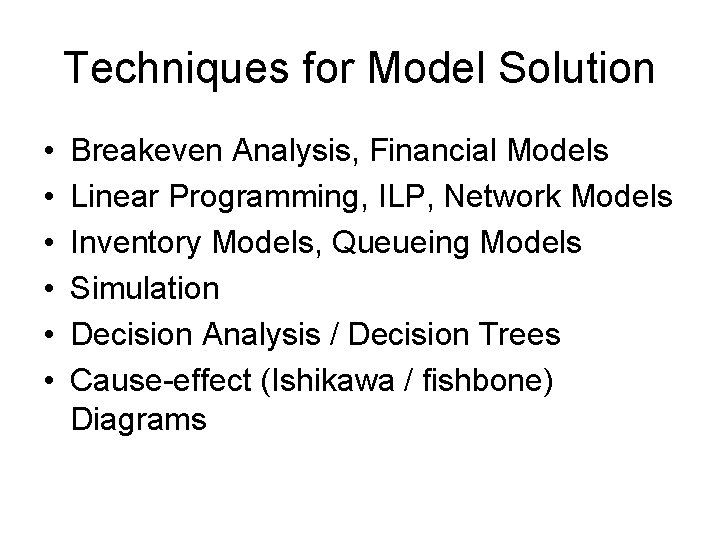Techniques for Model Solution • • • Breakeven Analysis, Financial Models Linear Programming, ILP, Network Models Inventory Models, Queueing Models Simulation Decision Analysis / Decision Trees Cause-effect (Ishikawa / fishbone) Diagrams